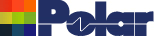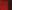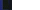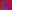Track Resistance Calculator – calculates DC series resistance of PCB tracks PCB trace resistance calculator option for Si9000e and Si8000m (former TRC100) Track Resistance Calculator is available as an option for the Si8000m and Si9000eWork in mils (thou), mm or micronsConvert between unitsChoose material resistivityIdeal for fine geometry tracksCustomize for other materialsCopy dimensions from Si9000e PCB Track resistance calculator Need to calculate PCB track resistance? The Si9000e PCB Track resistance calculator lets you enter track shape and length, along with material type, the trace resistance calculator will give you an answer in Ohms for the trace you describe or the transmission line geometries pasted from Si9000e. TRC100 is useful if you are working on fine geometries, and need to take care about series loss. You may also find TRC100 useful in power supply applications. (If you need to calculate PCB Impedance or insertion loss, please look at the Si9000e PCB transmission line design pages) How to measure track resistance AP145 How track resistance is calculated AP144 Calculating Impedance & insertion loss using a field solver Si9000e